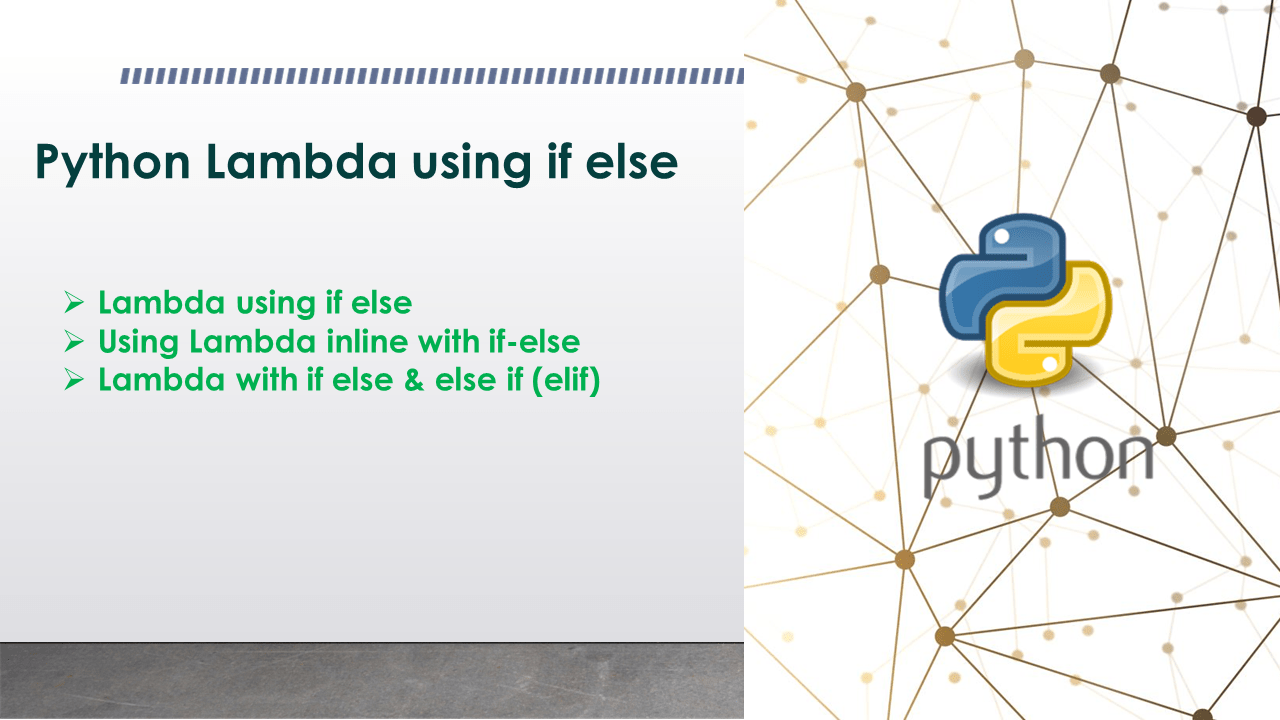# Python Lambda using if else

How to use an if else in Python lambda? You can use the if-else statement in a lambda as part of an expression. The lambda should have only one expression so here, it should be if-else. The if returns the body when the condition is satisfied, and the else body is returned when the condition is not satisfied. A Python lambda function is used to execute an anonymous function. This function can take any number of arguments, but can only have one expression and they can be used wherever function objects are required.

Note that the expression in the lambda function body should return some value. If the if-else expression does not return any value, the result from a lambda will be a `None` value.

## 1. Syntax of Lambda using if else

Following is the syntax of the using if-else statement on lambda expression.

``````
# Syntax
lambda arguments,[argument,arguments] : <statement1> if <condition> else <statement2>
``````

Here, statement1 is returned when if condition is True and statement2 when if condition is False.

## 2. Lambda using if else

You can use the if-else statement as part of the python lambda expression. after evaluating an expression make sure it returns a value for both satisfied and unsatisfied conditions. if no value is returned, it returns the None value.

``````
# Lambda function using if-else
min = lambda a, b : a if(a < b) else b
print(min(10, 20))

# Output:
# 10
``````

Here, the lambda function will return `a` when if condition is true and return `b` when if condition is false.

## 3. Using Lambda inline with if-else

The same example above can be written as an inline invocation. Here, we surround the lambda function with parentheses and place the values for the arguments next to it enclosed within parentheses.

``````
# Lambda function using if-else
min = (lambda a, b : a if(a < b) else b)(10,20)
print(min)

# Output:
# 10
``````

Here, the lambda function min takes two arguments and returns the minimum value of the arguments.

## 4. Lambda with if else & else if (elif)

You can also use the nested if else & else if statement in python lambda expression. Remember that the lambda expression can take only one expression hence, you need to write this nested if else in a single expression.

``````
# Lambda function using if else & else if
min = lambda a, b, c : f"{a} is smaller" if(a < b & b < c) \
else f"{b} is smaller"  if (b < c) else f"{c} is smaller"
print(min(40, 30, 10))

# Output:
# 10
``````

Here, we pass three numbers to the lambda function, by using a nested if else statement as an expression, we return the value that is the smallest of three values.

## Conclusion

In this article, you have learned different examples of using if else & else if in python lambda expression. You can use the if-else statement in a lambda as part of an expression. The lambda should have only one expression so here, it should be if-else. The if returns the body when the condition is satisfied, and the else body is returned when the condition is not satisfied.

## Related Articles

### Naveen

I am a Data Engineer with 20+ years of experience in transforming data into actionable insights. Over the years, I have honed my expertise in designing, implementing, and maintaining data pipelines with frameworks like Apache Spark, PySpark, Pandas, R, Hive and Machine Learning. My journey in the field of data engineering has been a continuous learning, innovation, and a strong commitment to data integrity. I have started this SparkByExamples.com to share my experiences with the data as I come across. You can learn more about me at LinkedIn